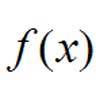#### You may also like### Towards Maclaurin

Build series for the sine and cosine functions by adding one term at a time, alternately making the approximation too big then too small but getting ever closer.### Building Approximations for Sin(x)

Build up the concept of the Taylor series### Taking Trigonometry Series-ly

Look at the advanced way of viewing sin and cos through their power series.

# What Do Functions Do for Tiny X?

##### Age 16 to 18Challenge Level

Archimedes, Bernoulli, Copernicus and de Moivre each try to think of a function which will be small near to the origin. Their choices are:

$$A(x) = \sin(x) \quad B(x) = 1 - \cos(x) \quad C(x) = \log(1+x) \quad D(x) = 1 - \frac{1}{1-x}$$

All of these functions equal zero when x is exactly zero, but the friends want to investigate how small their functions are when x is small but not exactly zero.

Use a spreadsheet to investigate these curves graphically for smaller and smaller values of x (you may like to try graphs with x ranging from -1 to 1, -0.1 to 0.1, -0.01 to 0.01 to begin with). Before you start, you might like to try to guess what will happen close to the origin in each case.

As you zoom in the scale what do you notice happening to the graphs in each case? What similarities do the functions have as we zoom in? What differences do they exhibit?

Can you predict the shapes of the graphs for x between -0.000001 and 0.000001? Test your predictions.

For each function can you write a simple polynomial approximation with 1 or 2 terms for the shape of each curve for very small values of x?